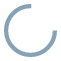### more

facts wiki news games kidztube appsIn math, the top part of a fraction is called the numerator. If the line part of the fraction is flat, the numerator is on top. If the line part of the fraction is at a slant, the numerator is on the left. The lower part is called the denominator of the fraction. For example:

• In 34 the numerator is "3".
• In 2376100 the numerator is "76".
• In (15+6)(35+7) the numerator is "(15+6)".
• In (a + b) / 6c, the numerator is "(a + b)".
view more...report a search problem